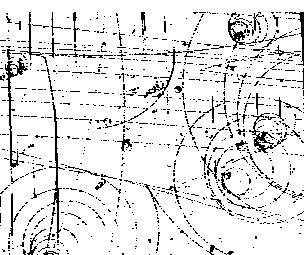# Yang-Mills Existence and Mass Gap Problem Yang-Mills Existence…Yang-Mills Existence and Mass Gap Problem

Yang-Mills Existence and Mass gap is an unsolved problem in mathematical physics. It’s one of the seven Millennium Prize Problems defined by the Clay Mathematics Institute with \$1million prize money for whoever solves it.

The problem is stated as follows:

Yang-Mills Existence and Mass Gap: Prove that for any compact single gauge group G, quantum Yang-Mills theory on R^4 exists and has a mass gap ∆ > 0 (Jaffe &Witten 141). A quantum field theory(QTF) such as Yang-Mills Theory is said to have a mass gap if the Hamiltonian H has no spectrum in the interval (0, ∆) for some ∆ > 0; the supremum of this ∆ is the mass m (Jaffe & Witten 142).

Yang-Mills Theory and the Wightman Axioms

In physics, the Wightman Axioms are mathematical formulae attempting to describe quantum field theory (QFT is what we use as the framework for creating quantum mechanical models of subatomic particles, treating matter and energy as excited states of the underlying physical field). There are 4 Axioms in total and the Millennium Prize Problem of Yang-Mills Existence and Mass Gap, Yang-Mills theory is proposed to satisfy them.

Mass Gap

If matter really is just “excitement’ in the underlying field, then there is a lowest energy level that matter can occupy. Mass gap is the difference between the vacuum that lowest energy level.

Quantum Yang-Mills theory
http://www.claymath.org/sites/default/files/yangmills.pdf

Yang-Mills Theory and the Mass Gap Problem
http://online.sfsu.edu/meredith/301/Papers/BordagarayFinalTerm.pdf

Report on the Status of the Yang-Mills Millenium Prize Problem http://www.claymath.org/sites/default/files/ym2.pdf